Anzeige

# 1_Basic Structure of Computers.pptx

2. Feb 2023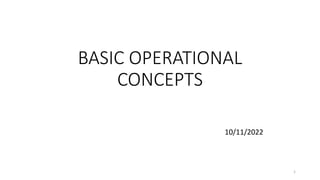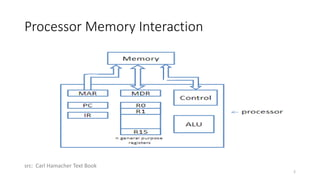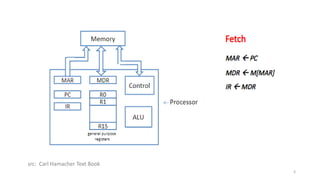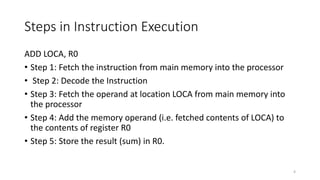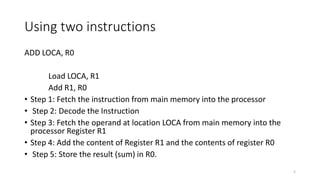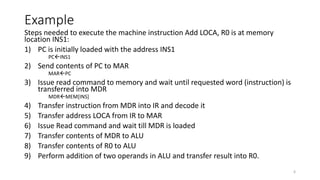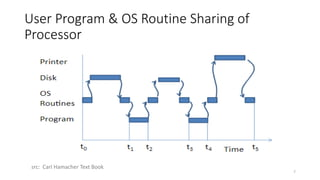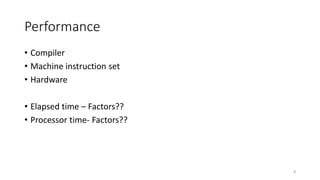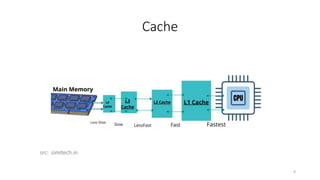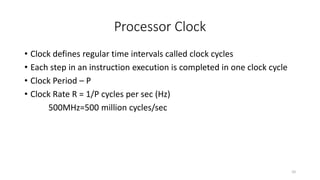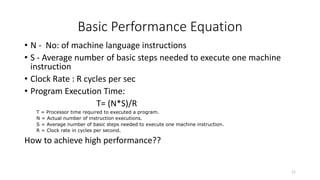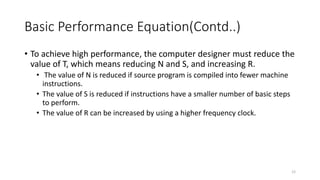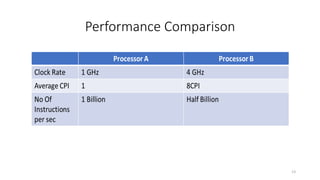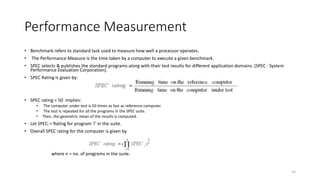1 von 14
Anzeige

### 1_Basic Structure of Computers.pptx

1. BASIC OPERATIONAL CONCEPTS 10/11/2022 1
2. Processor Memory Interaction 2 src: Carl Hamacher Text Book
3. 3 src: Carl Hamacher Text Book
4. Steps in Instruction Execution ADD LOCA, R0 • Step 1: Fetch the instruction from main memory into the processor • Step 2: Decode the Instruction • Step 3: Fetch the operand at location LOCA from main memory into the processor • Step 4: Add the memory operand (i.e. fetched contents of LOCA) to the contents of register R0 • Step 5: Store the result (sum) in R0. 4
5. Using two instructions ADD LOCA, R0 Load LOCA, R1 Add R1, R0 • Step 1: Fetch the instruction from main memory into the processor • Step 2: Decode the Instruction • Step 3: Fetch the operand at location LOCA from main memory into the processor Register R1 • Step 4: Add the content of Register R1 and the contents of register R0 • Step 5: Store the result (sum) in R0. 5
6. Example Steps needed to execute the machine instruction Add LOCA, R0 is at memory location INS1: 1) PC is initially loaded with the address INS1 PCINS1 2) Send contents of PC to MAR MARPC 3) Issue read command to memory and wait until requested word (instruction) is transferred into MDR MDRMEM[INS] 4) Transfer instruction from MDR into IR and decode it 5) Transfer address LOCA from IR to MAR 6) Issue Read command and wait till MDR is loaded 7) Transfer contents of MDR to ALU 8) Transfer contents of R0 to ALU 9) Perform addition of two operands in ALU and transfer result into R0. 6
7. User Program & OS Routine Sharing of Processor 7 src: Carl Hamacher Text Book
8. Performance • Compiler • Machine instruction set • Hardware • Elapsed time – Factors?? • Processor time- Factors?? 8
9. Cache 9 src: simitech.in
10. Processor Clock • Clock defines regular time intervals called clock cycles • Each step in an instruction execution is completed in one clock cycle • Clock Period – P • Clock Rate R = 1/P cycles per sec (Hz) 500MHz=500 million cycles/sec 10
11. Basic Performance Equation • N - No: of machine language instructions • S - Average number of basic steps needed to execute one machine instruction • Clock Rate : R cycles per sec • Program Execution Time: T= (N*S)/R T = Processor time required to executed a program. N = Actual number of instruction executions. S = Average number of basic steps needed to execute one machine instruction. R = Clock rate in cycles per second. How to achieve high performance?? 11
12. Basic Performance Equation(Contd..) • To achieve high performance, the computer designer must reduce the value of T, which means reducing N and S, and increasing R. • The value of N is reduced if source program is compiled into fewer machine instructions. • The value of S is reduced if instructions have a smaller number of basic steps to perform. • The value of R can be increased by using a higher frequency clock. 12
13. Performance Comparison 13
14. Performance Measurement • Benchmark refers to standard task used to measure how well a processor operates. • The Performance Measure is the time taken by a computer to execute a given benchmark. • SPEC selects & publishes the standard programs along with their test results for different application domains. (SPEC : System Performance Evaluation Corporation). • SPEC Rating is given by: • SPEC rating = 50 implies: • The computer under test is 50 times as fast as reference-computer. • The test is repeated for all the programs in the SPEC suite. • Then, the geometric mean of the results is computed. • Let SPECi = Rating for program ‘i’ in the suite. • Overall SPEC rating for the computer is given by where n = no. of programs in the suite. 14
Anzeige